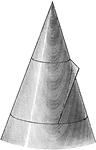### Conic Section Showing Parabola

Illustration showing the definition of a parabola as a conic section. "The section of a right circular…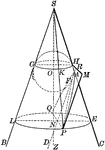### Conic Section Showing Parabola

Illustration showing the definition of a parabola as a conic section. "The section of a right circular…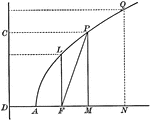### Parabola With Coordinates and Latus Rectum

Illustration of a parabola showing that any point of a parabola is the mean proportional between the…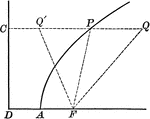### Point on Parabola

Illustration of a parabola showing point P is equidistant to the focus and the directrix.### Point on Parabola

Illustration of a parabola. "If a line PT is drawn from any point P of the curve, bisecting the angle…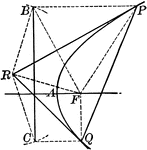### Tangent to a Parabola

Illustration of a tangent line drawn from an external point to a parabola.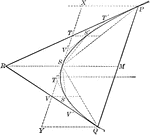### Tangents and Chords of a Parabola

Illustration showing that the area of a parabolic segment made by a chord is two thirds the area of…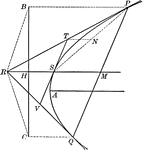### Tangents to a Parabola

Illustration showing that if two tangents RP and RQ are drawn from a point R to a parabola, the line…